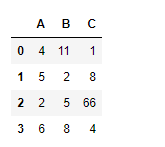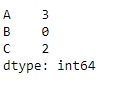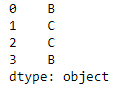# Python | Pandas dataframe.idxmax()

• Last Updated : 19 Nov, 2018

Python is a great language for doing data analysis, primarily because of the fantastic ecosystem of data-centric python packages. Pandas is one of those packages and makes importing and analyzing data much easier.

Pandas` dataframe.idxmax()` function returns index of first occurrence of maximum over requested axis. While finding the index of the maximum value across any index, all NA/null values are excluded.

Attention geek! Strengthen your foundations with the Python Programming Foundation Course and learn the basics.

To begin with, your interview preparations Enhance your Data Structures concepts with the Python DS Course. And to begin with your Machine Learning Journey, join the Machine Learning - Basic Level Course

Syntax: DataFrame.idxmax(axis=0, skipna=True)

Parameters :
axis : 0 or ‘index’ for row-wise, 1 or ‘columns’ for column-wise
skipna : Exclude NA/null values. If an entire row/column is NA, the result will be NA

Returns : idxmax : Series

Example #1: Use `idxmax()` function to function to find the index of the maximum value along the index axis.

 `# importing pandas as pd``import` `pandas as pd`` ` `# Creating the dataframe ``df ``=` `pd.DataFrame({``"A"``:[``4``, ``5``, ``2``, ``6``], ``                   ``"B"``:[``11``, ``2``, ``5``, ``8``],``                   ``"C"``:[``1``, ``8``, ``66``, ``4``]})`` ` `# Print the dataframe``df`Now apply the `idxmax()` function along the index axis.

 `# applying idxmax() function.``df.idxmax(axis ``=` `0``)`

Output :If we look at the values in the dataframe, we can verify the result returned by the function. The function returned a pandas series object containing the index of maximum value in each column.

Example #2: Use `idxmax()` function to find the index of the maximum value along the column axis. The dataframe contains `NA` values.

 `# importing pandas as pd``import` `pandas as pd`` ` `# Creating the dataframe ``df ``=` `pd.DataFrame({``"A"``:[``4``, ``5``, ``2``, ``None``],``                   ``"B"``:[``11``, ``2``, ``None``, ``8``], ``                   ``"C"``:[``1``, ``8``, ``66``, ``4``]})`` ` `# Skipna = True will skip all the Na values``# find maximum along column axis``df.idxmax(axis ``=` `1``, skipna ``=` `True``)`

Output :The output is a pandas series containing the column label for each row which has the maximum value.

My Personal Notes arrow_drop_up